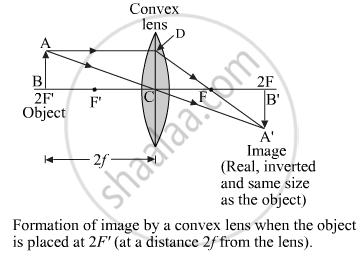Share

# An Object is Placed at a Distance Equal to 2f In Front of a Convex Lens. Draw a Labelled Ray Diagram to Show the Formation of Image. State Two Characteristics of the Image Formed. - CBSE Class 10 - Science

ConceptRefraction of Light Image Formation in Lenses Using Ray Diagrams - Convex Lens

#### Question

An object is placed at a distance equal to 2f in front of a convex lens. Draw a labelled ray diagram to show the formation of image. State two characteristics of the image formed.

#### Solution

Image formation diagram:Two characteristics of the image formed:
(1)Nature of image – real and inverted.
(2)Size of Image – same size as that of object​.

Is there an error in this question or solution?

#### APPEARS IN

Solution An Object is Placed at a Distance Equal to 2f In Front of a Convex Lens. Draw a Labelled Ray Diagram to Show the Formation of Image. State Two Characteristics of the Image Formed. Concept: Refraction of Light - Image Formation in Lenses Using Ray Diagrams - Convex Lens.
S